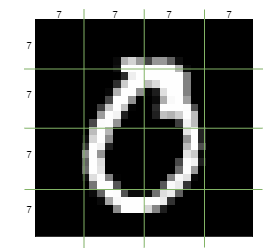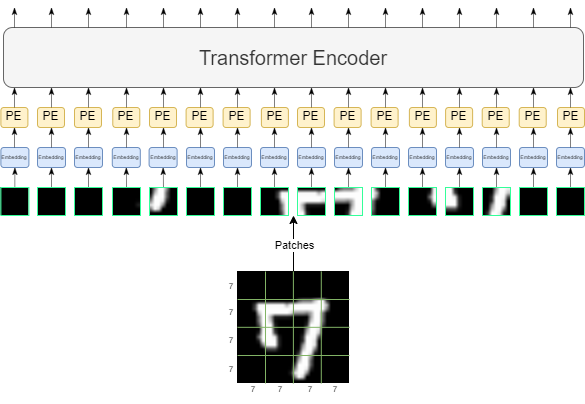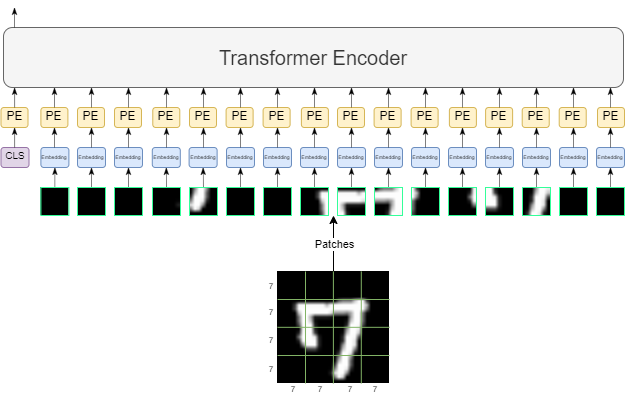In this blog post, we will learn about vision transformers (ViT), and implement an MNIST classifier with it. We will go step-by-step and understand every part of the vision transformers clearly, and you will see the motivations of the authors of the original paper in some of the parts of the architecture.

If you checked my previous blog post about implementing the transformers, it will be much easier to understand but if you already know about transformers, you can skip it.

We will only use the encoder part of the transformer architecture because we are not trying to generate anything, so encoder is all we need for now classification.

Let’s start very simple. We can try to use the transformers for images by framing the pixels as tokens, as we do in the NLP tasks, and train that way. But if you go and try this, you will notice that it will be very slow to train, because calculating the attention scores for any given sequence to transformers will take n2 operations. So even if you use MNIST images which are 28×28 pixels, it will require (28*28)2 operations, which is simply too much even for these very small images. So first we should find a solution to fix this problem.

Instead of framing each pixel as a token, we can divide our image to n patches, and think of each patch as a token. For example, if n is 4 and we are using 28×28 MNIST images, we can divide our image like this:And we can think of each of the patches as a token to feed into the network. This way instead of (28*28)2 operations, we have (4*4)2, which is much much easier.

In NLP, we need to convert each word into a numeric value(s), but we don’t have to do this with images since all pixels are already numeric values.

Next, we need to map each token into an embedding. Let’s say our embedding size will be 64. We have 7×7 sized patches and we can map them to a 64-sized embedding with a single fully connected layer.Now we have to add positional encoding to our embeddings since transformers have no concept of positions, and we know that the ordering of the image patches is important. We can do this by simply adding a learnable parameter to each patch and giving our network a chance to learn the positional encoding itself. We have 16 different image patches and each of them is 64-sized embedding vectors right now, so our positional encoding vector will also have the size 16×64, and we will simply sum them up.

We are almost done! We can now plug our data into the encoder part of the transformer. All these operations can be summarized with this figure:The encoder in the transformer will simply transform each input to a same-sized output. However, we are only interested in a single output at the end, which is the class of the image. So we should be selecting one of the 16 outputs. But we don’t want to just select a processed image patch’s output, we want something that considers all of them. To get an output like this, we can use an additional token.

We have a 16×64-sized matrix as our data after we did the embedding (before adding positional encodings), in which we framed each of the image patches as tokens. Now let’s consider an additional token called cls (stands for classification) token, so our matrix size will now be 17×64.

We will add this token to our sequence after the embedding step, and before the positional encoding step. Because we want to add positional encoding to this cls token as well. Now we feed our new 17×64-sized matrix to the encoder and get a 17×64-sized output. We want the transformed output of the cls token, so we will just take it and dump the rest.Now we have a 64-sized vector and all we want to do is map this 64-sized vector to 10 classes, which is easily achievable by using a fully connected layer!

Now we are done! The rest is only running the cross entropy loss and training our network.

Let’s start implementing this by creating the most important part, the architecture itself. We will use PyTorch’s implementation of transformer encoder for the transformer part. If you want to implement your own transformer from scratch you can check out my previous post.

import torch
import torch.nn.functional as F
from torch import nn
from torchvision import datasets, transforms

class ViT(nn.Module):
def __init__(self, img_width, img_channels, patch_size, d_model, num_heads, num_layers, num_classes, ff_dim):
super().__init__()

self.patch_size = patch_size

# given 7x7 flattened patch, map it into an embedding
self.patch_embedding = nn.Linear(img_channels * patch_size * patch_size, d_model)

# cls_token we are using we will be concatenating
self.cls_token = nn.Parameter(torch.randn(1, 1, d_model))

# (1, 4*4 + 1, 64)
# + 1 because we add cls tokens
self.position_embedding = nn.Parameter(
torch.rand(1, (img_width // patch_size) * (img_width // patch_size) + 1, d_model)
)

encoder_layer = nn.TransformerEncoderLayer(
)
self.transformer_encoder = nn.TransformerEncoder(encoder_layer, num_layers=num_layers)

# mapping 64 to 10 at the end
self.fc = nn.Linear(d_model, num_classes)

def forward(self, x):
N, C, H, W = x.shape

# we divide the image into 4 different 7x7 patches, and then flatten those patches
# img shape will be 4*4 x 7*7
x = x.unfold(2, self.patch_size, self.patch_size).unfold(3, self.patch_size, self.patch_size)
x = x.contiguous().view(N, C, -1, self.patch_size, self.patch_size)
x = x.permute(0, 2, 3, 4, 1).contiguous().view(N, -1, C * self.patch_size * self.patch_size)

# each 7*7 flatten patch will be embedded to 64 dim vector
x = self.patch_embedding(x)

# cls tokens concatenated after repeating it for the batch
cls_tokens = self.cls_token.repeat(N, 1, 1)
x = torch.cat((cls_tokens, x), dim=1)

x = x + self.position_embedding

# transformer takes 17x64 tensor, like it is a sequence with 17 words (17 because 4*4 + 1 from cls)
x = self.transformer_encoder(x)

# only taking the transformed output of the cls token
x = x[:, 0]

# mapping to number of classes
x = self.fc(x)

return x


We are done implementing the ViT model and now we will specify our hyperparameters and make our DataLoader ready:

batch_size = 128
lr = 3e-4
num_epochs = 15

img_width = 28
img_channels = 1
num_classes = 100
patch_size = 7
embedding_dim = 64
ff_dim = 2048
num_layers = 3
weight_decay = 1e-4

batch_size=batch_size,
shuffle=True,
)

batch_size=batch_size,
shuffle=True,
)


Time for defining our model and writing the training loop. Let’s evaluate our dataset at the end of every batch and write everything to TensorBoard so we can see our training process and validation results.

from torch.utils.tensorboard import SummaryWriter
from datetime import datetime

device = "cuda:0" if torch.cuda.is_available() else "cpu"
print(f"{device=}")

model = ViT(
img_width=img_width,
img_channels=img_channels,
patch_size=patch_size,
d_model=embedding_dim,
num_layers=num_layers,
num_classes=num_classes,
ff_dim=ff_dim,
).to(device)

writer = SummaryWriter(f"runs/vit-mnist_{datetime.now().strftime('%Y-%m-%d_%H:%M:%S')}")

for epoch in range(num_epochs):
losses = []
total_train = 0
correct_train = 0

model.train()
img = img.to(device)
label = label.to(device)

pred = model(img)
loss = F.cross_entropy(pred, label)

pred_class = torch.argmax(pred, dim=1)
correct_train += (pred_class == label).sum().item()
total_train += pred.shape

loss.backward()
optimizer.step()

losses.append(loss.item())

writer.add_scalar("train acc", correct_train / total_train, epoch)

model.eval()
total = 0
correct = 0